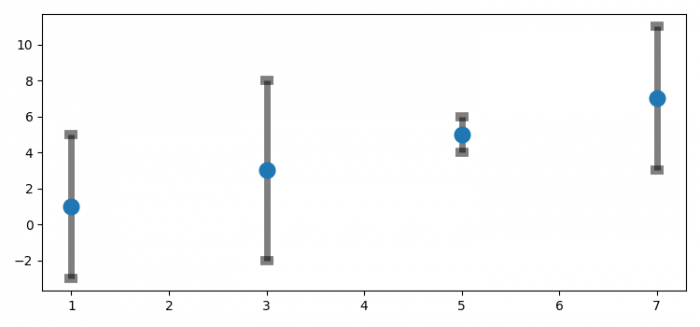# Transparent error bars without affecting the markers in Matplotlib

To make transparent error bars without affecting markers in matplotlib, we can take the following steps −

## Steps

• Set the figure size and adjust the padding between and around the subplots.

• Make lists x, y and z for data.

• Initialize a variable error_bar_width=5

• Plot y versus x as lines and/or markers with attached errorbars.

• Set the alpha value of bars and caps.

• To display the figure, use show() method.

## Example

from matplotlib import pyplot as plt

plt.rcParams["figure.figsize"] = [7.50, 3.50]
plt.rcParams["figure.autolayout"] = True

x = [1, 3, 5, 7]
y = [1, 3, 5, 7]
z = [4, 5, 1, 4]

error_bar_width = 5

markers, caps, bars = plt.errorbar(x, y, z, capsize=5, elinewidth=error_bar_width,
markeredgewidth=7, fmt='o', ecolor='black', capthick=2)
[bar.set_alpha(0.5) for bar in bars]
[cap.set_alpha(0.5) for cap in caps]

plt.show()

## Output

It will produce the following output −